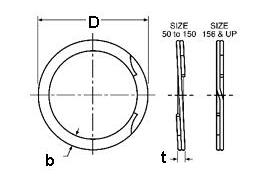RR-885

Display Units: inches |  metric
Request Quick Quote for this part.
 Ring Specs (D) Free Diameter: 9.151 +0.065 / -0.000 in. (t) Thickness: 0.086 +0.004 / -0.004 in. (b) Radial Wall: 0.375 +0.004 / -0.008 in. Groove Specs: (B) Application Diameter: 8.858 in. (G) Groove Diameter: 9.080 +0.008 / -0.008 in. (W) Groove Width: 0.094 +0.006 / -0.000 in. Groove Depth: 0.111 in. Other Specs Approximate Weight per 1000: (m) Material Thickness: 0.042 in. (N) Ring Number of Turns: 2.000 (Pg) Theoretical Thrust Load Capacity - Groove Yield:Notes: Yield Strength of Groove Material (Ys): 45,000 psi. Calculated using a safety factor (K) of 2Equation:Pg = [ B * d * Ys * pi ] / K    = [ (8.858 in.) * (0.111 in.) * (45,000 psi) * 3.14 ] / 2 69,465 lbs. (Pr) Theoretical Thrust Load Capacity - Ring Shear:Notes: Ring Material: Carbon Spring Steel (SAE 1070-1090). Shear Strength of Ring Material (Ss): 126,000 psi. Calculated using a safety factor (K) of 3Equation:Pr = [ B * t * Ss * pi ] / K    = [ (8.858 in.) * (0.086 in.) * (126,000 psi) * 3.14 ] / 3 100,464 lbs. Industry Equivalent Part Number(s):RR-885, KM-885, WH-885